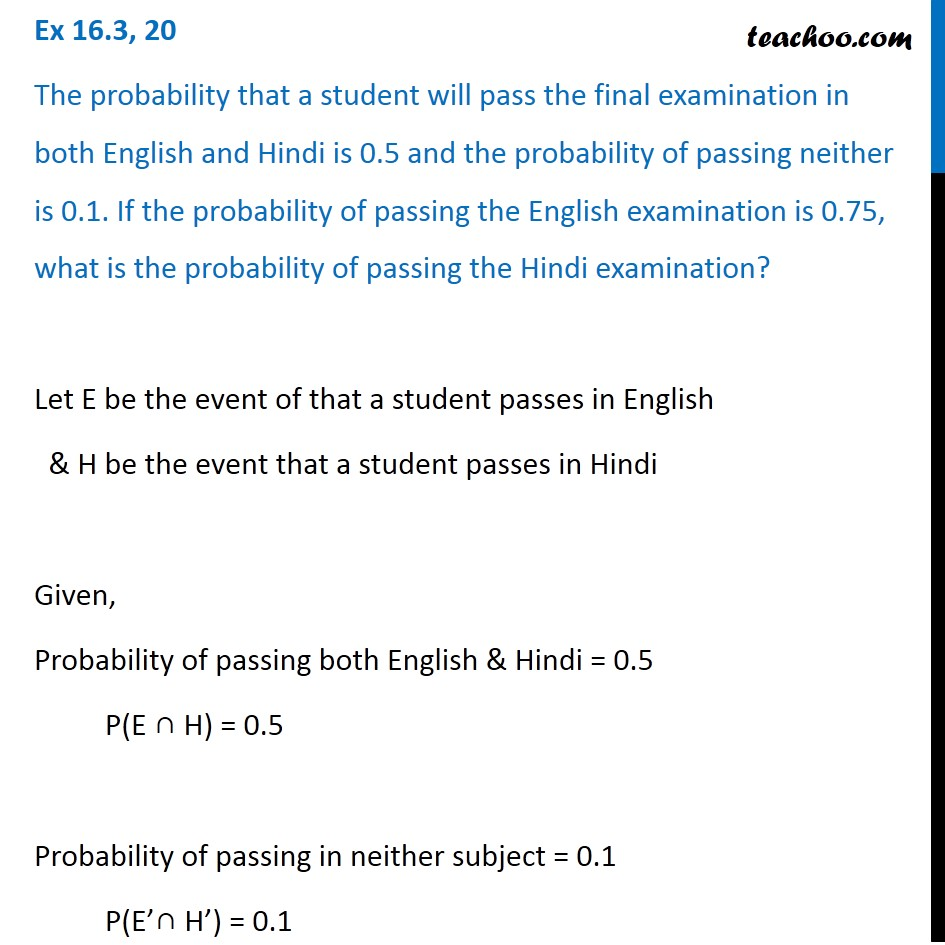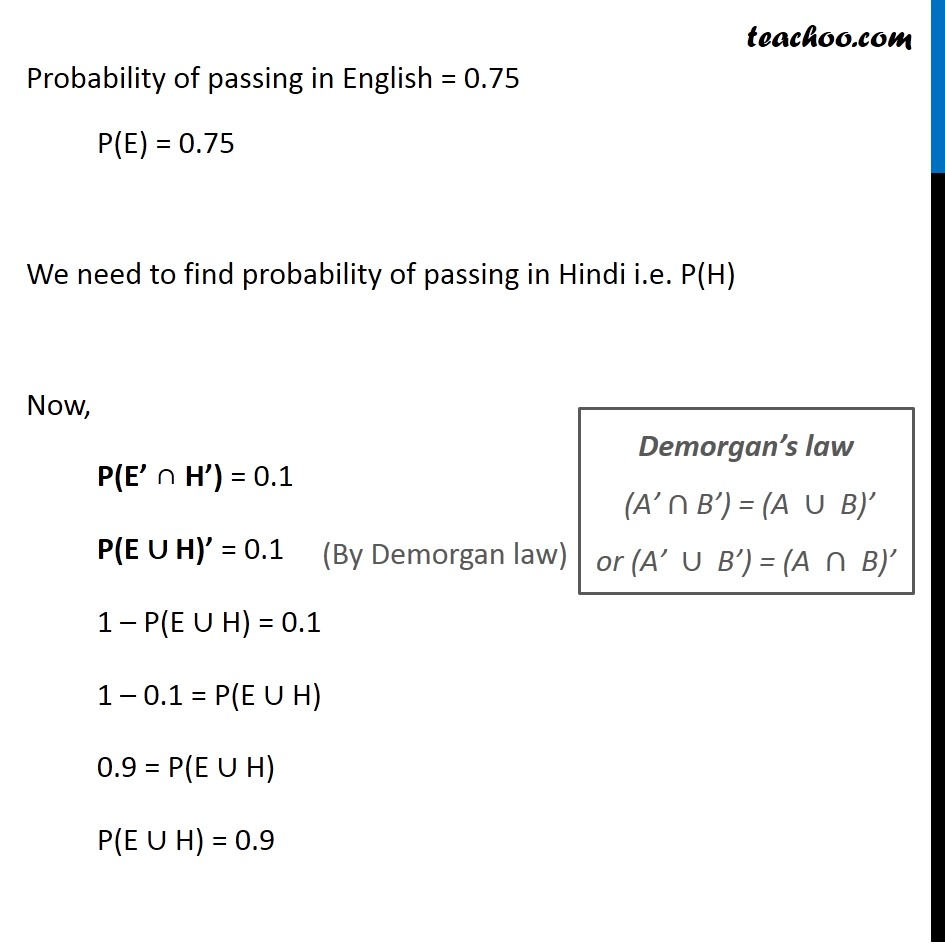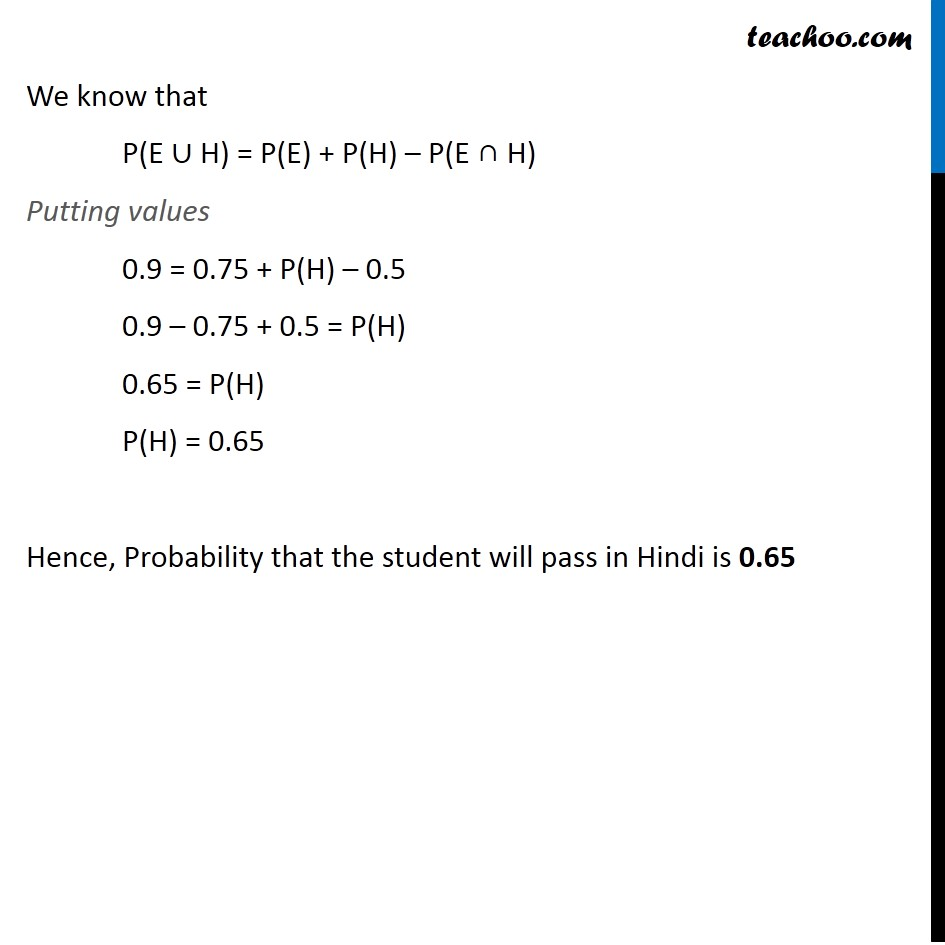Ex 14.2

Chapter 14 Class 11 Probability
Serial order wiseLearn in your speed, with individual attention - Teachoo Maths 1-on-1 Class

### Transcript

Ex 14.2, 20 The probability that a student will pass the final examination in both English and Hindi is 0.5 and the probability of passing neither is 0.1. If the probability of passing the English examination is 0.75, what is the probability of passing the Hindi examination? Let E be the event of that a student passes in English & H be the event that a student passes in Hindi Given, Probability of passing both English & Hindi = 0.5 P(E ∩ H) = 0.5 Probability of passing in neither subject = 0.1 P(E’∩ H’) = 0.1 Probability of passing in English = 0.75 P(E) = 0.75 We need to find probability of passing in Hindi i.e. P(H) Now, P(E’ ∩ H’) = 0.1 P(E ∪ H)’ = 0.1 1 – P(E ∪ H) = 0.1 1 – 0.1 = P(E ∪ H) 0.9 = P(E ∪ H) P(E ∪ H) = 0.9 Demorgan’s law "(A’"∩"B’) = (A "∪" B)’" "or (A’ "∪" B’) = (A "∩" B)’" We know that P(E ∪ H) = P(E) + P(H) – P(E ∩ H) Putting values 0.9 = 0.75 + P(H) – 0.5 0.9 – 0.75 + 0.5 = P(H) 0.65 = P(H) P(H) = 0.65 Hence, Probability that the student will pass in Hindi is 0.65